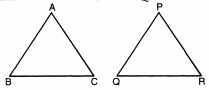# If area of 2similar triangles are ...

If area of 2similar triangles are equal then prove that these triangles are congruent

Sia 🤖 9 months, 3 weeks ago

Given:
{tex}∆ABC\sim∆PQR{/tex}
and {tex}ar∆ABC=ar∆PQR{/tex}To prove: {tex}∆ABC\cong∆PQR{/tex}
Proof: {tex}∆ABC\sim∆PQR{/tex}
Also {tex}\operatorname { ar } ( \Delta A B C ) = \operatorname { ar } ( \Delta P Q R ){/tex} (given)
or, {tex}\frac { \operatorname { ar } ( \Delta A B C ) } { \operatorname { ar } ( \Delta P Q R ) } = 1{/tex}
Or {tex}\frac{AB^2}{PQ^2}=\frac{BC^2}{QR^2}=\frac{CA^2}{RP^2}=1{/tex}
Or {tex}\frac{AB}{PQ}=\frac{BC}{QR}=\frac{CA}{RP}=1{/tex}
Hence we get that
AB=PQ,BC=QR and CA=RP
Hence {tex}∆ABC\cong ∆PQR{/tex}

Related Questions:

Subscribe complete study pack and get unlimited access to selected subjects. Effective cost is only ₹ 12.5/- per subject per month. Based on the latest CBSE & NCERT syllabus.## myCBSEguide

Trusted by 70 Lakh Students

#### CBSE Test Generator

Create papers in minutes

Print with your name & Logo

3 Lakhs+ Questions

Solutions Included

Based on CBSE Blueprint

Best fit for Schools & Tutors

#### Work from Home

• Work from home with us
• Create questions or review them from home

No software required, no contract to sign. Simply apply as teacher, take eligibility test and start working with us. Required desktop or laptop with internet connection

﻿